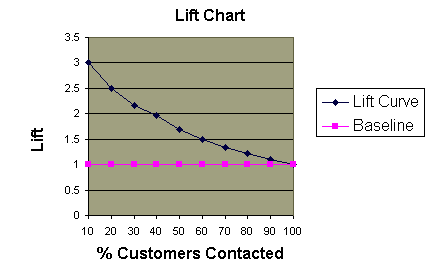• Lift is a measure of the effectiveness of a predictive model calculated as the ratio between the results obtained with and without the predictive model.
• Cumulative gains and lift charts are visual aids for measuring model performance
• Both charts consist of a lift curve and a baseline
• The greater the area between the lift curve and the baseline, the better the model

### Example Problem 1

A company wants to do a mail marketing campaign. It costs the company \$1 for each item mailed. They have information on 100,000 customers. Create a cumulative gains and a lift chart from the following data.
• Overall Response Rate: If we assume we have no model other than the prediction of the overall response rate, then we can predict the number of positive responses as a fraction of the total customers contacted. Suppose the response rate is 20%. If all 100,000 customers are contacted we will receive around 20,000 positive responses.
Cost (\$) Total Customers Contacted Positive Responses
100000
100000
20000
• Prediction of Response Model: A response model predicts who will respond to a marketing campaign. If we have a response model, we can make more detailed predictions. For example, we use the response model to assign a score to all 100,000 customers and predict the results of contacting only the top 10,000 customers, the top 20,000 customers, etc.
Cost (\$) Total Customers Contacted Positive Responses
10000
10000
6000
20000
20000
10000
30000
30000
13000
40000
40000
15800
50000
50000
17000
60000
60000
18000
70000
70000
18800
80000
80000
19400
90000
90000
19800
100000
100000
20000

Cumulative Gains Chart:

• The y-axis shows the percentage of positive responses. This is a percentage of the total possible positive responses (20,000 as the overall response rate shows).
• The x-axis shows the percentage of customers contacted, which is a fraction of the 100,000 total customers.
• Baseline (overall response rate): If we contact X% of customers then we will receive X% of the total positive responses.
• Lift Curve: Using the predictions of the response model, calculate the percentage of positive responses for the percent of customers contacted and map these points to create the lift curve.Lift Chart:

• Shows the actual lift.
• To plot the chart: Calculate the points on the lift curve by determining the ratio between the result predicted by our model and the result using no model.
• Example: For contacting 10% of customers, using no model we should get 10% of responders and using the given model we should get 30% of responders. The y-value of the lift curve at 10% is 30 / 10 = 3.Analyzing the Charts: Cumulative gains and lift charts are a graphical representation of the advantage of using a predictive model to choose which customers to contact. The lift chart shows how much more likely we are to receive respondents than if we contact a random sample of customers. For example, by contacting only 10% of customers based on the predictive model we will reach 3 times as many respondents as if we use no model.

### Evaluating a Predictive Model

We can assess the value of a predictive model by using the model to score a set of customers and then contacting them in this order. The actual response rates are recorded for each cutoff point, such as the first 10% contacted, the first 20% contacted, etc. We create cumulative gains and lift charts using the actual response rates to see how much the predictive model would have helped in this situation. The information can be used to determine whether we should use this model or one similar to it in the future.

### Example Problem 2

Using the response model P(x)=100-AGE(x) for customer x and the data table shown below, construct the cumulative gains and lift charts. Ties in ranking should be arbitrarily broken by assigning a higher rank to who appears first in the table.

 Customer Name Height Age Actual Response Alan 70 39 N Bob 72 21 Y Jessica 65 25 Y Elizabeth 62 30 Y Hilary 67 19 Y Fred 69 48 N Alex 65 12 Y Margot 63 51 N Sean 71 65 Y Chris 73 42 N Philip 75 20 Y Catherine 70 23 N Amy 69 13 N Erin 68 35 Y Trent 72 55 N Preston 68 25 N John 64 76 N Nancy 64 24 Y Kim 72 31 N Laura 62 29 Y

1.  Calculate P(x) for each person x

2.  Order the people according to rank P(x)

 Customer Name P(x) Actual Response Alex 88 Y Amy 87 N Hilary 81 Y Philip 80 Y Bob 79 Y Catherine 77 N Nancy 76 Y Jessica 75 Y Preston 75 N Laura 71 Y Elizabeth 70 Y Kim 69 N Erin 65 Y Alan 61 N Chris 58 N Fred 52 N Margot 49 N Trent 45 N Sean 35 Y John 24 N
3.  Calculate the percentage of total responses for each cutoff point
• Response Rate = Number of Responses  / Total Number of Responses (10)
 Total Customers Contacted Number of Responses Response Rate 2 1 10% 4 3 30% 6 4 40% 8 6 60% 10 7 70% 12 8 80% 14 9 90% 16 9 90% 18 9 90% 20 10 100%
4.  Create the cumulative gains chart:
• The lift curve and the baseline have the same values for 10%-20% and 90%-100%.5.  Create the lift chart: